Examples

Chapter 7 Class 11 Permutations and Combinations (Term 2)
Serial order wise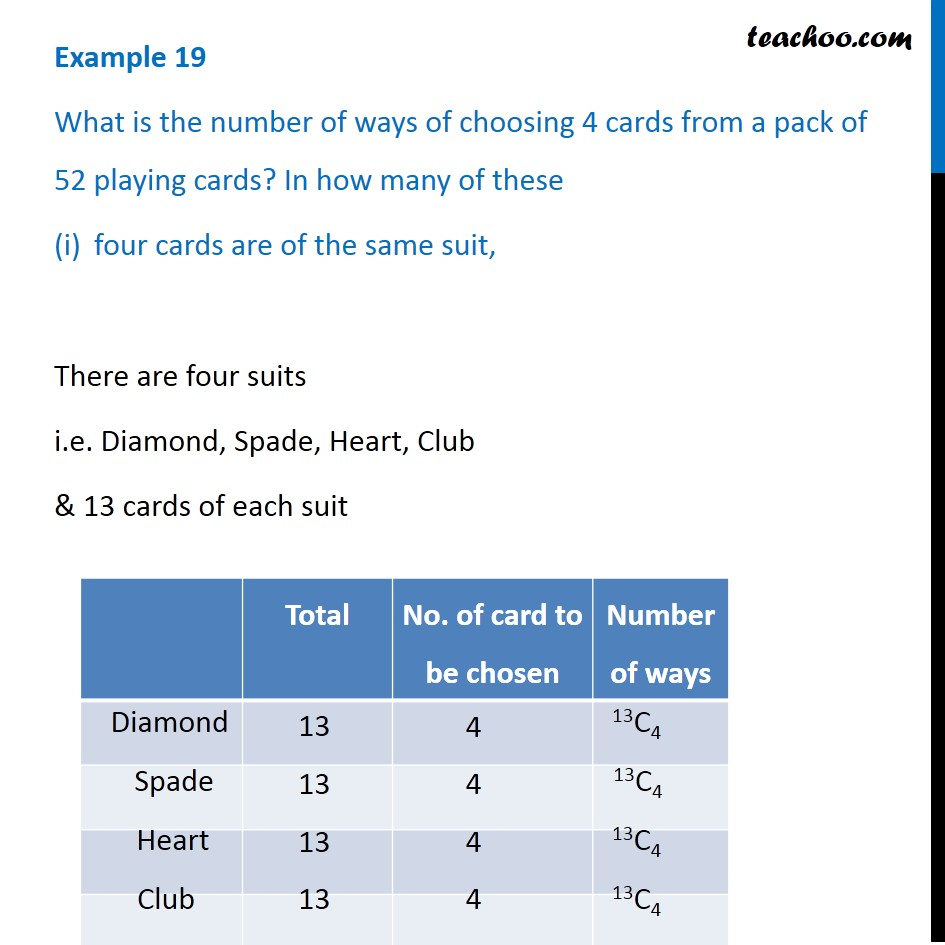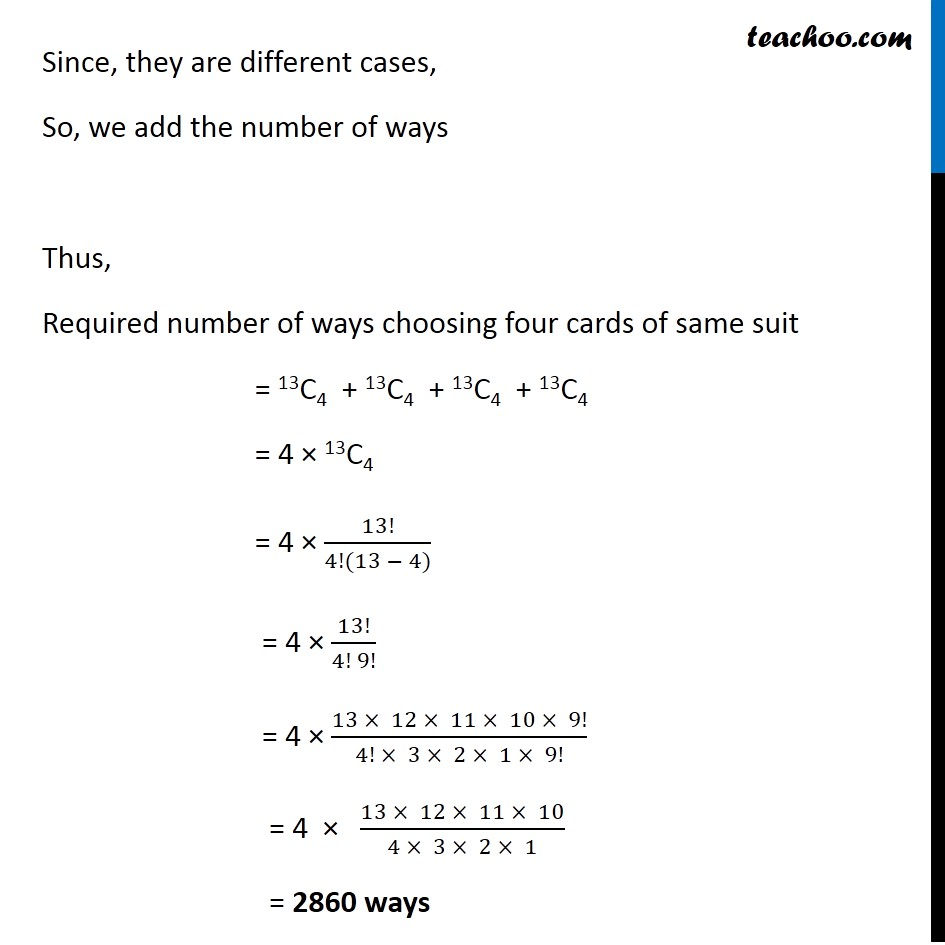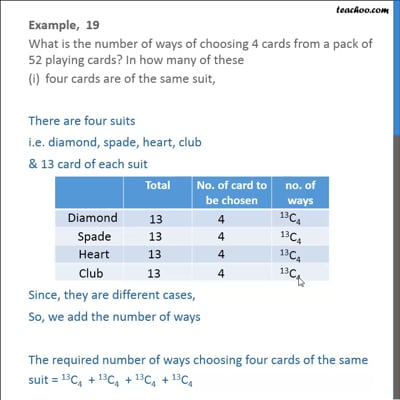This video is only available for Teachoo black users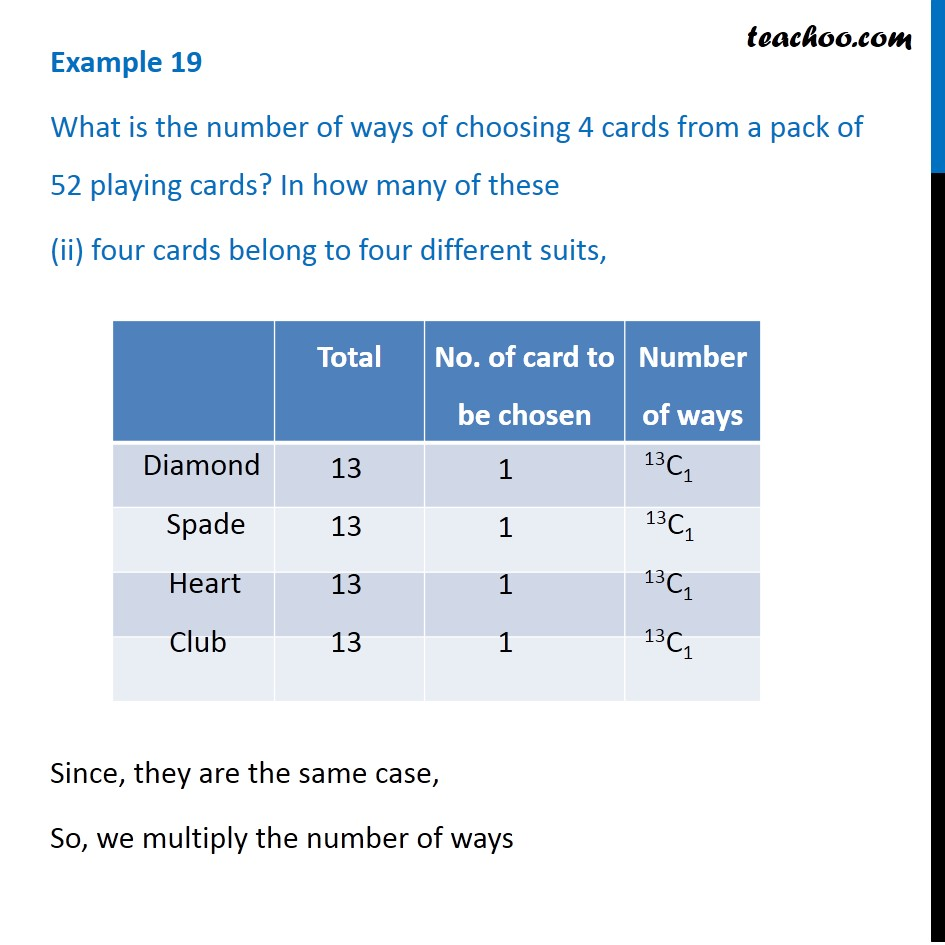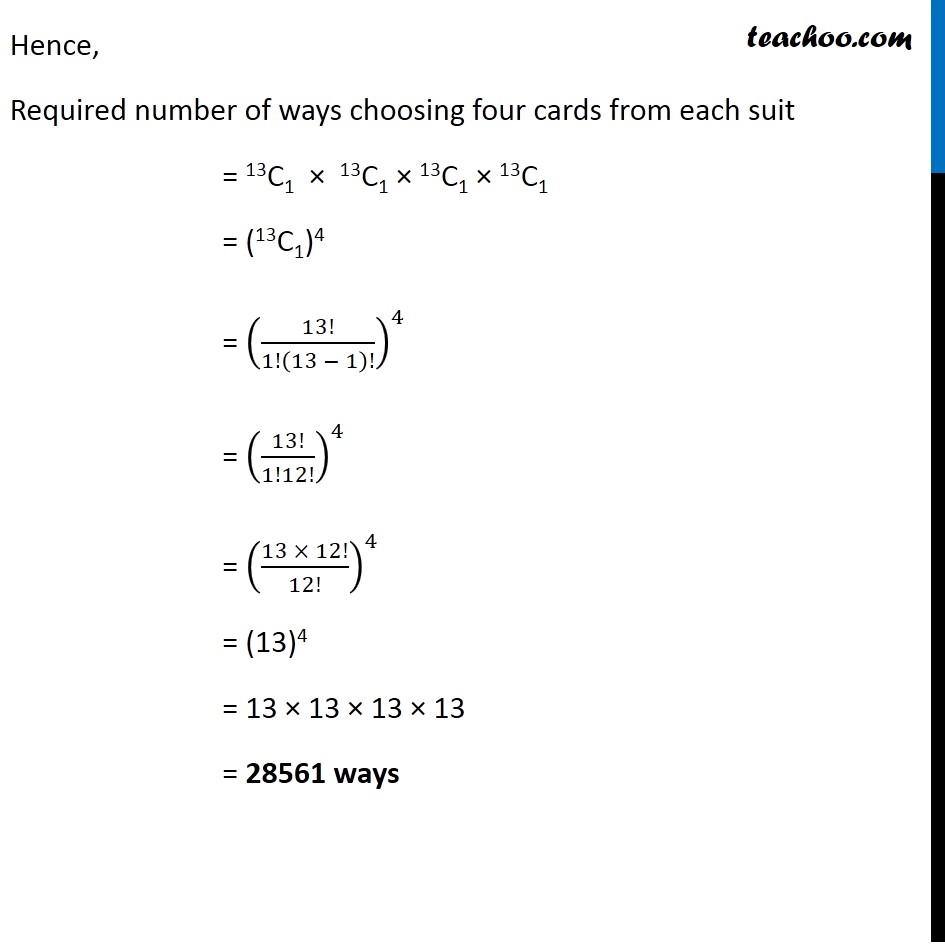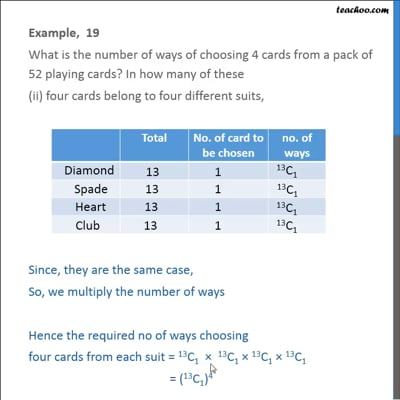This video is only available for Teachoo black users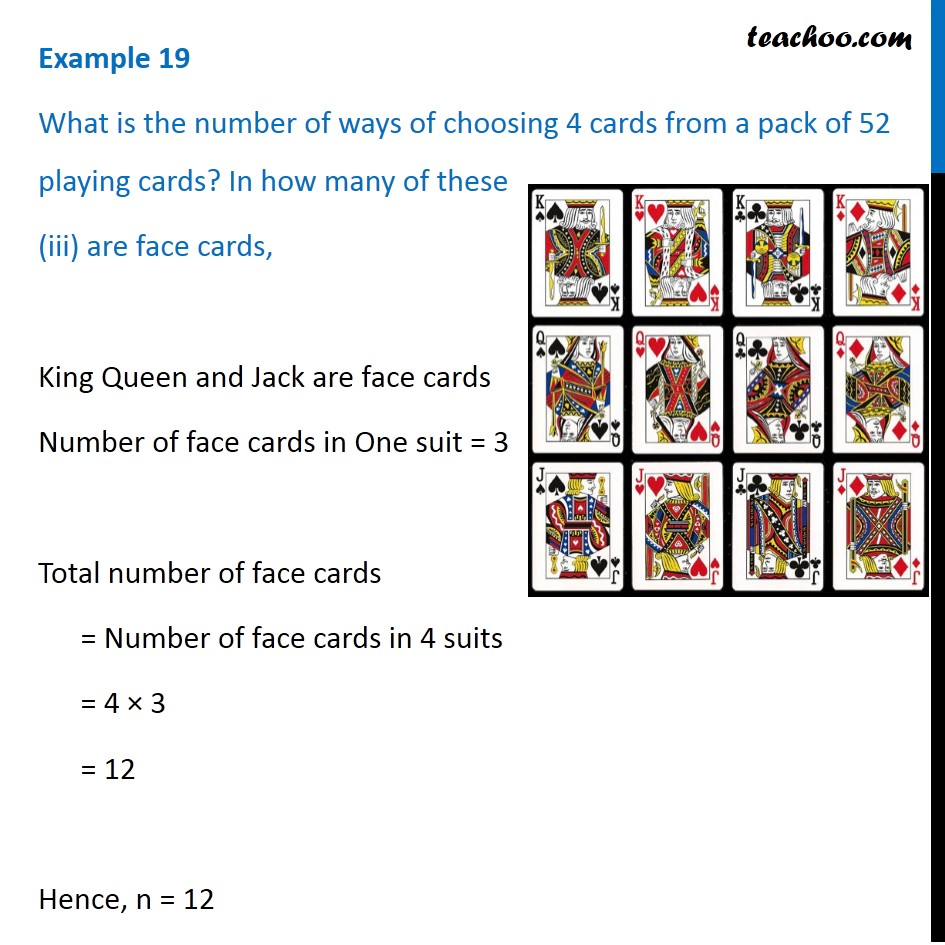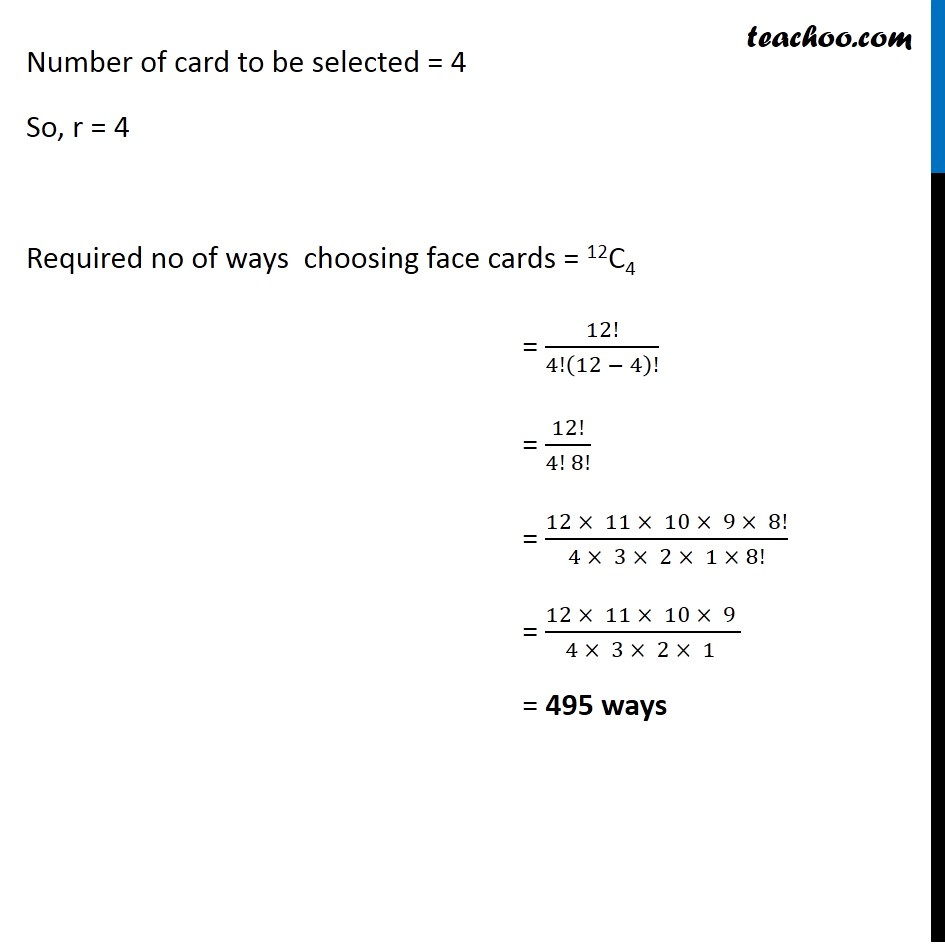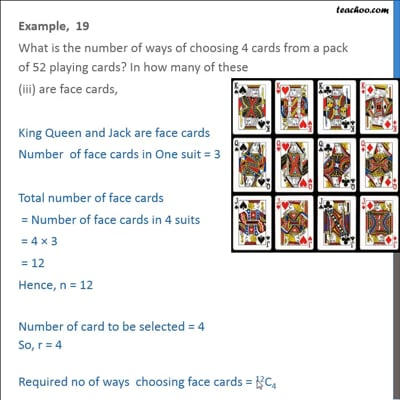This video is only available for Teachoo black users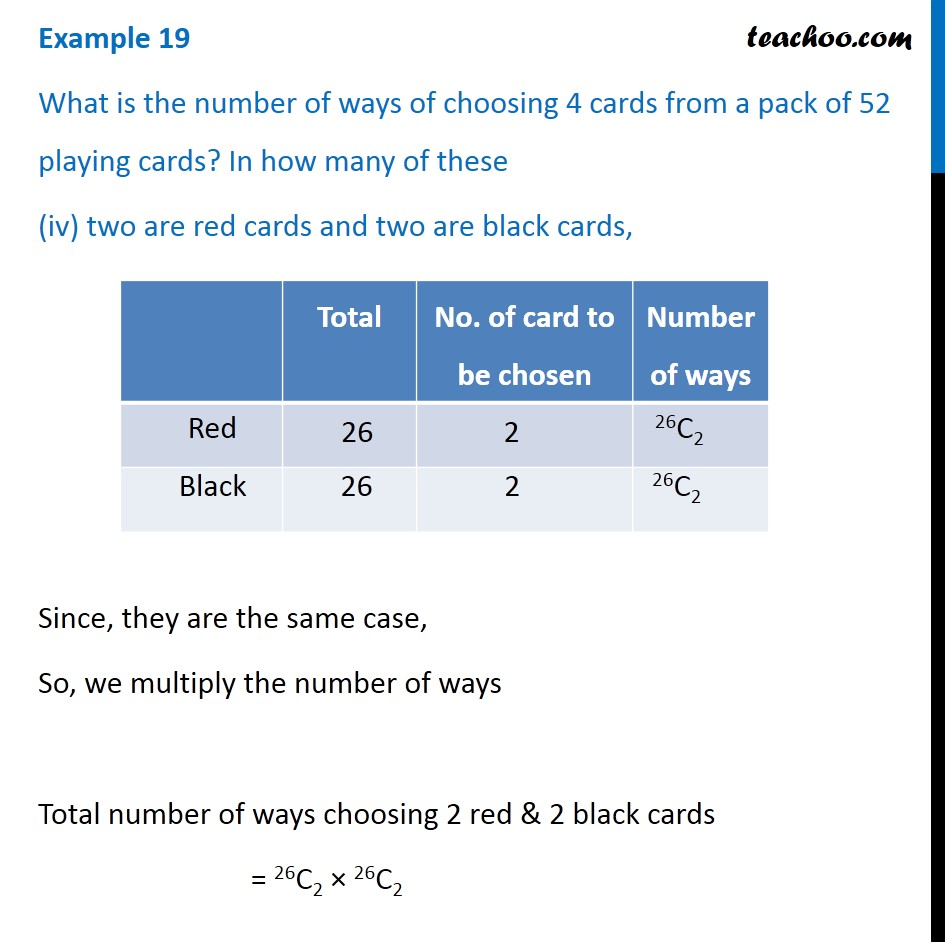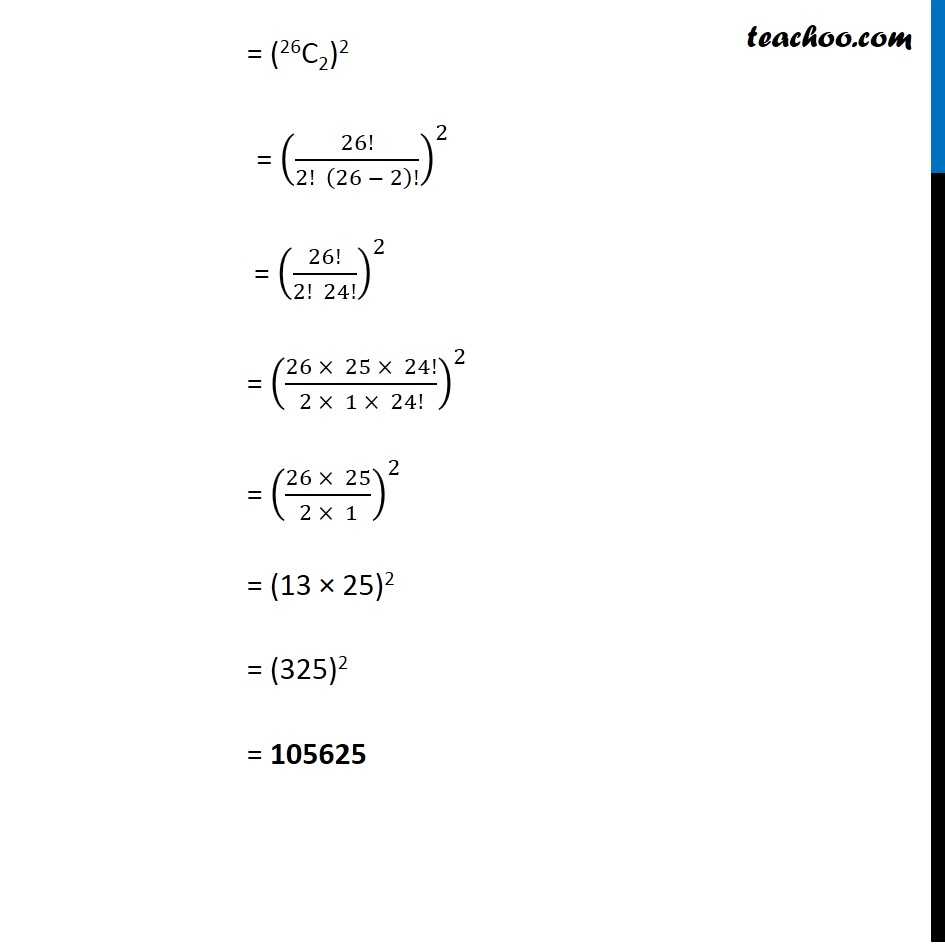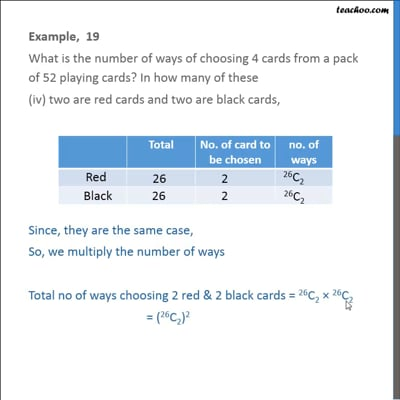This video is only available for Teachoo black users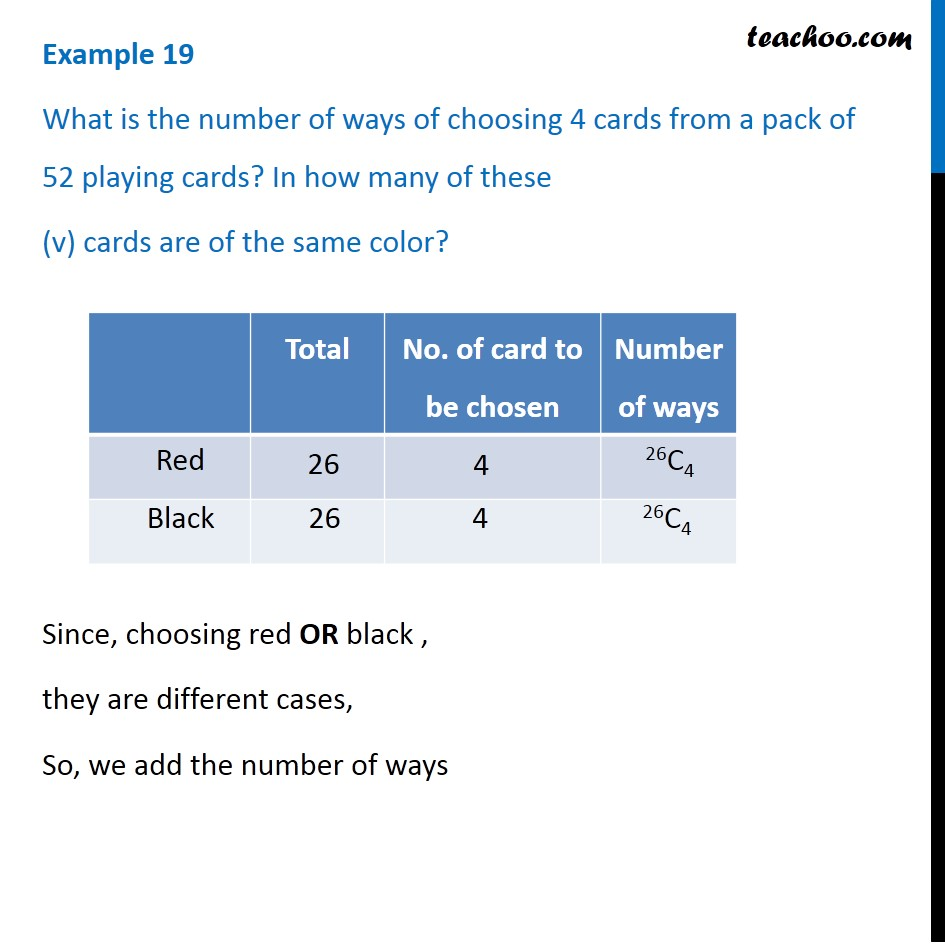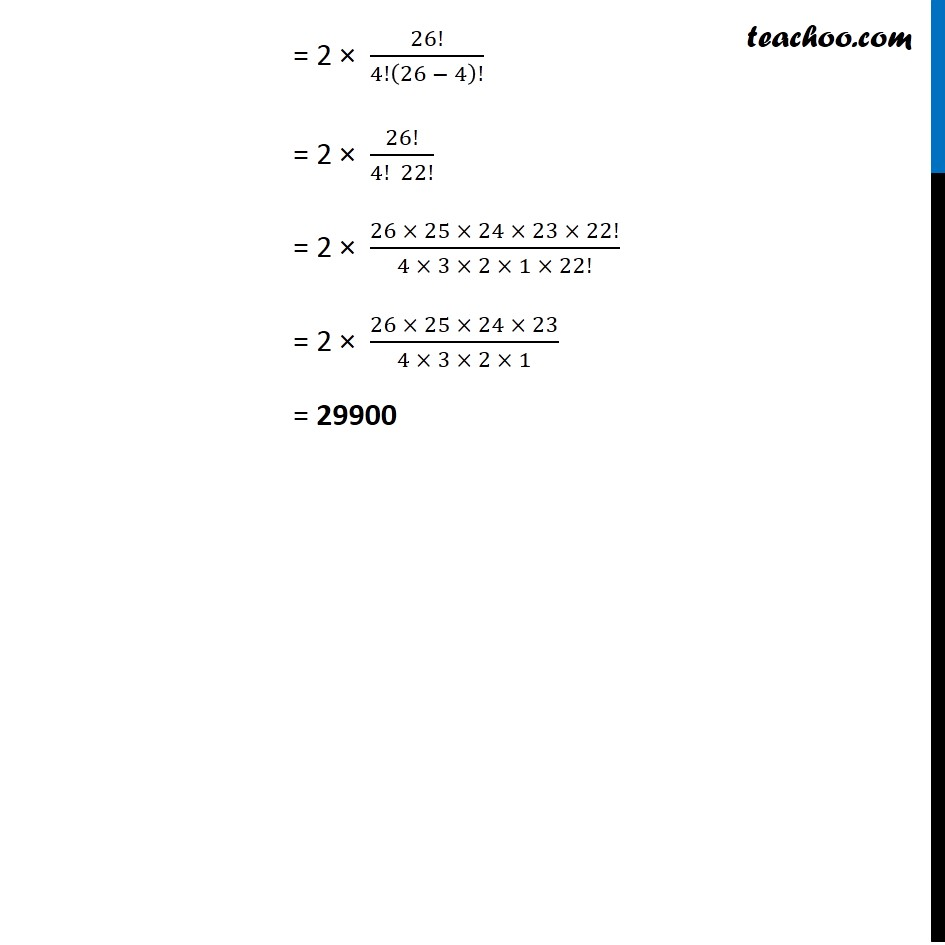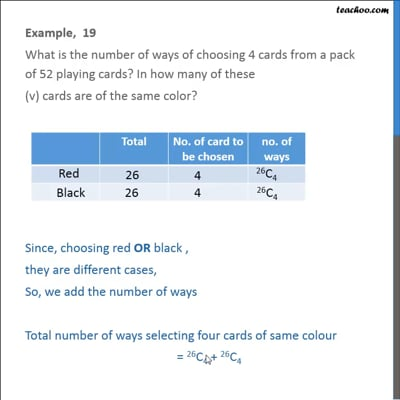This video is only available for Teachoo black users

### Transcript

Example 19 What is the number of ways of choosing 4 cards from a pack of 52 playing cards? In how many of these four cards are of the same suit, There are four suits i.e. Diamond, Spade, Heart, Club & 13 cards of each suit Since, they are different cases, So, we add the number of ways Thus, Required number of ways choosing four cards of same suit = 13C4 + 13C4 + 13C4 + 13C4 = 4 × 13C4 = 4 × 13!/(4!(13 − 4)) = 4 × 13!/(4! 9!) = 4 × (13 × 12 × 11 × 10 × 9!)/(4! × 3 × 2 × 1 × 9!) = 4 × (13 × 12 × 11 × 10)/(4 × 3 × 2 × 1) = 2860 ways Example 19 What is the number of ways of choosing 4 cards from a pack of 52 playing cards? In how many of these (ii) four cards belong to four different suits, Since, they are the same case, So, we multiply the number of ways Hence, Required number of ways choosing four cards from each suit = 13C1 × 13C1 × 13C1 × 13C1 = (13C1)4 = (13!/1!(13 − 1)!)^4 = (13!/1!12!)^4 = ((13 × 12!)/12!)^4 = (13)4 = 13 × 13 × 13 × 13 = 28561 ways Example 19 What is the number of ways of choosing 4 cards from a pack of 52 playing cards? In how many of these (iii) are face cards, King Queen and Jack are face cards Number of face cards in One suit = 3 Total number of face cards = Number of face cards in 4 suits = 4 × 3 = 12 Hence, n = 12 Number of card to be selected = 4 So, r = 4 Required no of ways choosing face cards = 12C4 = 12!/4!(12 − 4)! = 12!/(4! 8!) = (12 × 11 × 10 × 9 × 8!)/(4 × 3 × 2 × 1 × 8!) = (12 × 11 × 10 × 9 )/(4 × 3 × 2 × 1 ) = 495 ways Example 19 What is the number of ways of choosing 4 cards from a pack of 52 playing cards? In how many of these (iv) two are red cards and two are black cards, Since, they are the same case, So, we multiply the number of ways Total number of ways choosing 2 red & 2 black cards = 26C2 × 26C2 = (26C2)2 = (26!/(2! (26 − 2)!))^2 = (26!/(2! 24!))^2 = ((26 × 25 × 24!)/(2 × 1 × 24!))^2 = ((26 × 25)/(2 × 1))^2 = (13 × 25)2 = (325)2 = 105625 Example 19 What is the number of ways of choosing 4 cards from a pack of 52 playing cards? In how many of these (v) cards are of the same color? Since, choosing red OR black , they are different cases, So, we add the number of ways = 2 × 26!/4!(26 − 4)! = 2 × 26!/(4! 22!) = 2 × (26 × 25 × 24 × 23 × 22!)/(4 × 3 × 2 × 1 × 22!) = 2 × (26 × 25 × 24 × 23)/(4 × 3 × 2 × 1) = 29900